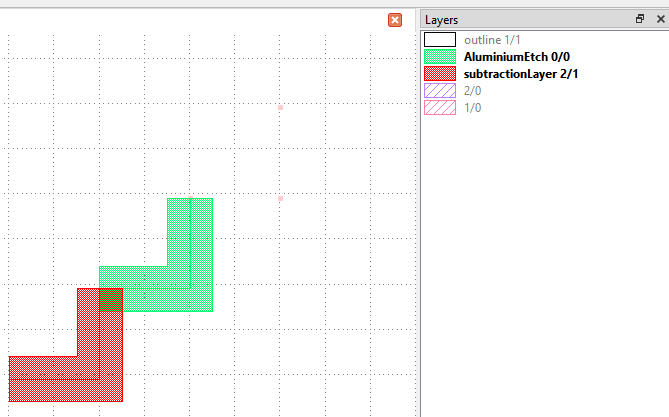# Dealing with layers in Python PCell

edited October 11

I have not managed to understand how layers are indexed in KLayout. The following code:
def produce_impl(self):

``````# This is the main part of the implementation: create the layout
view = pya.Application.instance().main_window().current_view()
layout = view.cellview(0).layout()
idxs =layout.layer_indexes()
li = layout.layer_infos()

print("layer indexes:", idxs)
print("layer infos", li)

for k,l in enumerate(idxs):
pts = [pya.Point(k/self.layout.dbu,k/self.layout.dbu),
pya.Point((1+k)/self.layout.dbu,k/self.layout.dbu),
pya.Point((1+k)/self.layout.dbu,(1+k)/self.layout.dbu)]
# create the shape
self.cell.shapes(l).insert(pya.Path(pts, 0.5/self.layout.dbu))
``````

gives output:

``````layer indexes: [0, 1, 2, 4, 5]
layer infos [<pya.LayerInfo object at 0x000000003146b768>, <pya.LayerInfo object at 0x000000003146bcc0>, <pya.LayerInfo object at 0x000000003146ba60>, <pya.LayerInfo object at 0x000000003146bdf0>, <pya.LayerInfo object at 0x000000003146be88>]
``````

But adding a PCell from the code produce the following:Which is not what I would have expected. Why is there no shape in every layer? And seems the order is not of the layers from layout.layer_indexes().

What am I doing wrong? Thanks

• You cannot access layers from the application from a PCell. Instead, the PCell will give you a layout object which is not the application's layout.

PCell layer parameters are given as LayerInfo structures. But the PCell helper class will provide the layer index as a service. This happens in the following way: declare a parameter with a name - e.g. "wheretogo". If the PCell helper class will see a parameter declared as Layer, is will provide the corresponding layer index in an attribute called like the parameter name plus "_layer". In the example that will be "wheretogo_layer".

Here is the code from the Python PCell sample:

``````    # parameter declaration as "Layer" with name "l" in __init__:
self.param("l", self.TypeLayer, "Layer", default = pya.LayerInfo(1, 0))
...
# in "produce_impl" "self.l_layer" is the layer index
self.cell().shapes(self.l_layer).insert(pya.Polygon(...))
``````

You can achieve the same by obtaining the layer index from the PCell's context layout:

``````    # in "produce_impl" you can turn self.l (a LayerInfo object) into a layer index:
layer_index = self.layout.layer(self.l)
``````

Matthias

• Thank you for your reply @Matthias. Unfortunately, I am not sure I understand. You wrote I cannot access layers from the PCell, but I can get a list of all layer indexes. Is it only that I cannot assign to them? The thing I want to do is to create a "fully parametric" class of PCell's. I have code that looks like this:

``````class VParser:
def __init__(self, filename):
g = {"sqrt": math.sqrt}
self.l = {}
with open(filename) as file:
``````

and filename is a python executable where I want to store variables, e.g. `a = 5`. In `produce_impl`I then want to

``````     exec("x = "+self.w_str, {}, vp.l)
w_dbu = vp.l['x']/self.layout.dbu
``````

where now `vp.l` is a `VParser` object.

With this construction, we can have other things than numbers setting e.g. sizes of objects. I can for example do

``````self.param("w_str", self.TypeString, "Width", default = "w")
``````

to have the width of something be w which is defined in a file. I can even do

``````self.param("w_str", self.TypeString, "Width", default = "w+a")
``````

if I want that.

Now, the issue is that I would like to be able to choose also the layer as a parameter in the external file. But I cannot understand how to do that. Perhaps it is not possible?

• Honestly, this is weird, but I guess I cannot convince you not to do that. I feels like delegating an architecture problem to a configuration. I rarely have seen such things doing good. In my experience such a scheme just adds obfuscation at the virtual benefit of some apparent flexibility. And I'm not talking about the error handling which you have to do or the inherent security holes you generate with "eval" on an input string.

But well ...

Anyway, if you want to introduce layers, treat them as strings - e.g "1/0" for layer 1, datatype 0. You can turn this string into a layer index you use:

``````layer_string = ...  # e.g. "1/0"
layer_index = self.layout.layer(pya.LayerInfo.from_string(layer_info))
``````

Matthias